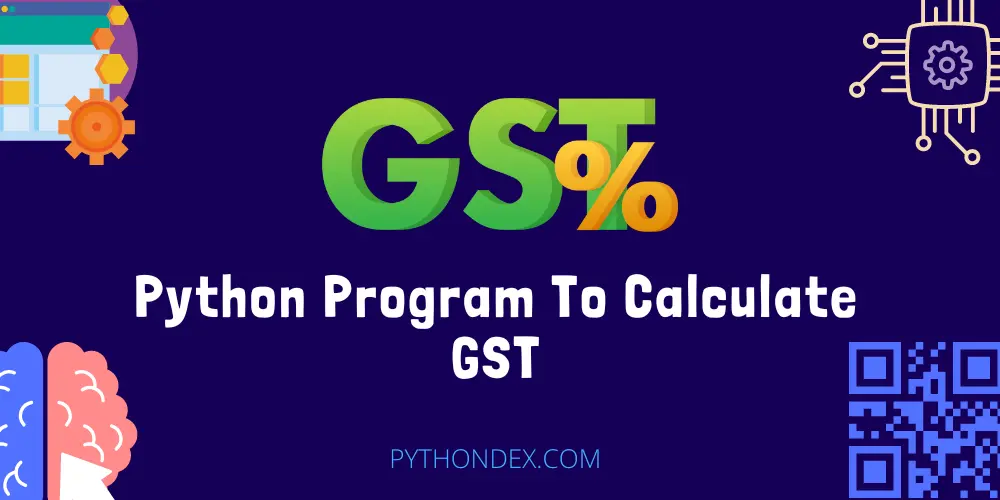ExplorePython Programs# Python Program To Calculate GSTWant to know how to create GST calculator program in python, then you are at the right place today in this tutorial. I will show you how to create python program to calculate GST with source code.

GST is goods and service tax it is applicable in India it is paid by the customers to the government so I have decided to created this tutorial to calculate how much GST you are paying.

## GST Calculator Python Code

``````
selling_price = int(input("Enter selling price of the product: "))
gst_rate = 18
gst_price = selling_price * 18 / 100
net_price = selling_price + gst_price
print(f"GST applied rs: {gst_price} at GST rate {gst_rate}%")
print(f"Total cost of the product after applying GST = {net_price}")
```
```

Above is the python program to calculate GST. Now to run this program you need to have python installed on your computer, If you don’t have then follow this guide: Install and setup python on your computer.

To run this python program, follow the below steps:

• Create a new folder for this python project.
• Open it in a code editor of your choice.
• Create a python file with an ending .py extension.
• Copy the above code and paste it in your file.
• Open a terminal or CMD at folder location
• And type this command python filename.py (Name of your file).

After running this program you will see that it will ask you to enter the selling price of the product then it will print the GST applied amount and net price price of the product below is an example output.

``````
Enter selling price of the product: 1000
GST applied rs: 180.0 at GST rate 18%
Total cost of the product after applying GST = 1180.0
```
```

As you can see we successfully created this program in python. Don’t want to create all the files and folders then you can run this program now using this online python compiler.

## Summary

This was a simple GST calculator program in python. I hope you found this tutorial helpful and useful. Do share this tutorial with your friends who might need this program.

Here are some more python program tutorials for you:

I hope you found what you were looking for from this tutorial, and if you want more python programs and tutorials like this, do join our Telegram channel for future updates.

Thanks for reading, have a nice day 🙂